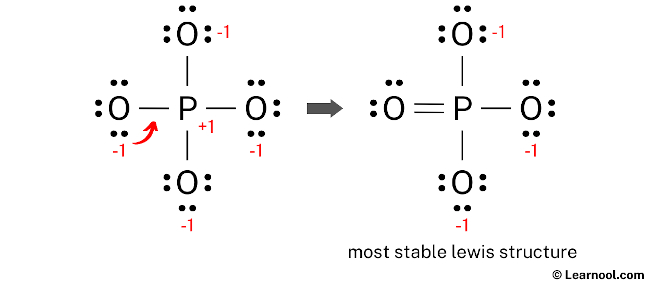# PO43- Lewis structure

PO43- (phosphate) has one phosphorus atom and four oxygen atoms.

In the PO43- Lewis structure, there is one double bond and three single bonds around the phosphorus atom, with four oxygen atoms attached to it. One oxygen atom with a double bond has two lone pairs, and three oxygen atoms with single bonds have three lone pairs.

Also, there is a negative (-1) charge on the three oxygen atoms with single bonds.

Contents

## Steps

To properly draw the PO43- Lewis structure, follow these steps:

#1 Draw a rough sketch of the structure
#2 Next, indicate lone pairs on the atoms
#3 Indicate formal charges on the atoms, if necessary
#4 Minimize formal charges by converting lone pairs of the atoms
#5 Repeat step 4 if necessary, until all charges are minimized

Let’s break down each step in more detail.

### #1 Draw a rough sketch of the structure

• First, determine the total number of valence electrons

In the periodic table, phosphorus lies in group 15, and oxygen lies in group 16.

Hence, phosphorus has five valence electrons and oxygen has six valence electrons.

Since PO43- has one phosphorus atom and four oxygen atoms, so…

Valence electrons of one phosphorus atom = 5 × 1 = 5
Valence electrons of four oxygen atoms = 6 × 4 = 24

Now the PO43- has a negative (-3) charge, so we have to add three more electrons.

So the total valence electrons = 5 + 24 + 3 = 32

• Second, find the total electron pairs

We have a total of 32 valence electrons. And when we divide this value by two, we get the value of total electron pairs.

Total electron pairs = total valence electrons ÷ 2

So the total electron pairs = 32 ÷ 2 = 16

• Third, determine the central atom

We have to place the least electronegative atom at the center.

Since phosphorus is less electronegative than oxygen, assume that the central atom is phosphorus.

Therefore, place phosphorus in the center and oxygens on either side.

• And finally, draw the rough sketch

### #2 Next, indicate lone pairs on the atoms

Here, we have a total of 16 electron pairs. And four P — O bonds are already marked. So we have to only mark the remaining twelve electron pairs as lone pairs on the sketch.

Also remember that phosphorus is a period 3 element, so it can keep more than 8 electrons in its last shell. And oxygen is a period 2 element, so it can not keep more than 8 electrons in its last shell.

Always start to mark the lone pairs from outside atoms. Here, the outside atoms are oxygens.

So for each oxygen, there are three lone pairs, and for phosphorus, there is zero lone pair because all twelve electron pairs are over.

Mark the lone pairs on the sketch as follows:

### #3 Indicate formal charges on the atoms, if necessary

Use the following formula to calculate the formal charges on atoms:

Formal charge = valence electrons – nonbonding electrons – ½ bonding electrons

For phosphorus atom, formal charge = 5 – 0 – ½ (8) = +1

For each oxygen atom, formal charge = 6 – 6 – ½ (2) = -1

Here, both phosphorus and oxygen atoms have charges, so mark them on the sketch as follows:

The above structure is not a stable Lewis structure because both phosphorus and oxygen atoms have charges. Therefore, reduce the charges (as below) by converting lone pairs to bonds.

### #4 Minimize formal charges by converting lone pairs of the atoms

Convert a lone pair of the oxygen atom to make a new P — O bond with the phosphorus atom as follows:Lone pair of left oxygen is converted, and got the most stable Lewis structure of PO43-

In the above structure, you can see that the central atom (phosphorus) forms an octet. And the outside atoms (oxygens) also form an octet. Hence, the octet rule is satisfied.

Now there is still a negative (-1) charge on the three oxygen atoms.

This is okay, because the structure with a negative charge on the most electronegative atom is the best Lewis structure. And in this case, the most electronegative element is oxygen.

Also, the above structure is more stable than the previous structures. Therefore, this structure is the most stable Lewis structure of PO43-.

And since the PO43- has a negative (-3) charge, mention that charge on the Lewis structure by drawing brackets as follows: